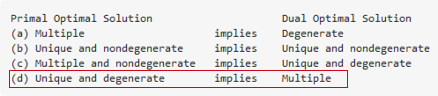•Gurobi Staff

Hi Michael,

I am not quite sure if I understand your question correctly.
A basic solution is called degenerate if one of the basic variables takes 0 value, thus you could just check whether your solution point has 0 values.

You say, you would like to get the reduced costs of all other optimal solutions, but a simplex algorithms returns exactly one optimal solution. If you are solving a MIP, please note that reduced costs are not defined for MIPs as described in our knowledge base article on Pi values.

Best regards,
Jaromił

•Hi Jaromił,

thanks a lot for your answer. I might have formulated it a bit ambiguous, sorry for that. I am talking about the case of a unique and degenerate primal solution with multiple dual optimal solutions, as seen in this graphic:(The table is from Sierksma's Linear and Integer Programming: Theory and Practice, Volume 1, page 144.)

Yes, checking for 0 value of the basic variables is an option to determine degeneracy but does a specific query for this exist as well? One which returns directly whether the primal solution is degenerate or not by using GRBModel.Get(xyz) for instance?

In case of multiple dual optimal solutions, the reduced costs for my primal optimal solution depend on the dual optimal solution chosen by the solver (at least that is my understanding). I was wondering whether it is possible to extract the reduced costs corresponding to all other dual optimal solutions as well?

Best regards,

Michael

•Gurobi Staff

Hi Michael,

I understand now, thank you for clarifying.

There is currently no specific way to query the degeneracy information other then checking the solution values "by hand".

Yes, in case of multiple dual solutions, you will obtain the reduced costs for your primal depending on the dual solution chosen by the solver. It is not possible to obtain the reduced costs for all dual solutions in such situations. Please note that obtaining all dual solutions may not even be possible in some cases as there can be an infinite number of them, see, e.g., the example found here.

Best regards,
Jaromił

•Gurobi Staff

You may also try to solve the LP with the barrier (method = 2) while switching the crossover phase off (crossover = 0).  The barrier algorithm will try to find a maximal cardinality solution, so if such exists in case (d) that you have described, you should be seeing more non-zeros in the dual solution.  Note, the above would of course be dependent on your problem behaving well from a numerical stand-point (crossover would try to fix some issues, if they occur), and it may be easier if you have LP in the "standard equality" primal-dual form, e.g.,

(P) min c'x: Ax = b, x>= 0

(D) max b'y: A'y+s = c, s >= 0

If this sounds obscure, you may want to try to read up on maximal cardinality property for the (path-following) interior point methods for LP.